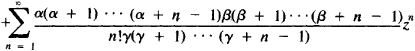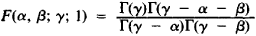# Hypergeometric Series

(redirected from Generalized Hypergeometric Functions)

## hypergeometric series

[‚hī·pər‚jē·ə′me·trik ′sir·ēz]
(mathematics)
A particular infinite series which in certain cases is a solution to the hypergeometric equation, and having the form:

## Hypergeometric Series

a series of the form

F(α, β; γ; z) = 1The hypergeometric series was studied for the first time by L. Euler in 1778. Expansions of many functions into infinite series are special cases of hypergeometric series. For example,The hypergeometric series has meaning if γ is not equal to zero or a negative integer. It converges at |z| < 1. If, in addition, γ - α - β > 0, then the hypergeometric series also converges for z = 1. In this case, Gauss’ formula is valid:where Γ(z) is the gamma function. An analytic function, defined for |z| < 1 by a hypergeometric series is called a hypergeometric function and plays an important role in the theory of differential equations.

References in periodicals archive ?
He begins by discussing generalized hypergeometric functions, of which Bessel functions are a special case, then applies the results to many useful representation of Bessel functions and their integrals by specializing the parameters.
Srivastava, Classes of analytic functions associated with the generalized hypergeometric functions, Appl.
Srivastava, Generalized hypergeometric functions associate with k-uniformly convex functions, Comput.
Srivastava, Certain subclasses of analytic functions associated with the generalized hypergeometric functions, Integral Transform Spec.
Srivastava: Univalent and starlike generalized hypergeometric functions, Canad.
He also develops Mellin transform techniques used to evaluate said integrals analytically and express the results either as infinite series or as sums of generalized hypergeometric functions.
In Section 4, starlikeness conditions of the Liu-Srivastava operator involving the generalized hypergeometric functions are investigated.
Srivastava, Generalized Hypergeometric functions associated with k-uniformly convex functions, Comput.
Srivastava,: Univalent and starlike generalized hypergeometric functions, Canad.
7), we take a = a' = c = c' = 1, s = 1, d = d' = b = b'= n = n' = 0 and further reduce both the multivariable H-functions to the generalized hypergeometric functions in the usual way, we get the expressions obtained by Goyal, Jain and Gaur [6, pp.

Site: Follow: Share:
Open / Close